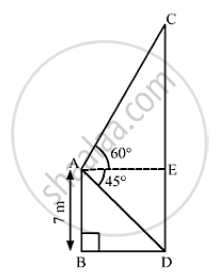# From the Top of a 7 Meter High Building, the Angle of Elevation of the Top of a Cable Tower is 60° and the Angle of Depression of Its Foot is 45° . Determine the Height of the Tower. - Mathematics

From the top of a 7 meter high building, the angle of elevation of the top of a cable tower is 60° and the angle of depression of its foot is 45° . Determine the height of the tower.

#### SolutionLet AB be the 7-m high building and CD be the cable tower,
We have,
AB = 7m, ∠CAE = 60°,∠DAE = ∠ADB = 45°
Also, DE = AB = 7m
In  ΔABD,

 tan 45° = (AB)/(BD)

⇒ 1= 7/(BD)

⇒  BD = 7 m

So, AE = BD = 7m
Also, in ΔACE,

 tan 60° = (CF)/(AE)

⇒  sqrt(3) = (CE) /7

⇒ CE = 7 sqrt(3) m

Now, CD = CE  +DE

= 7 sqrt(3) +7

= 7 ( sqrt(3) +1) m

= 7(1.732 +1)

= 7(2.732)

= 19.124

~~ 19.12m

So, the height of the tower is 19.12m.

Concept: Heights and Distances
Is there an error in this question or solution?
Chapter 14: Height and Distance - Exercises

#### APPEARS IN

RS Aggarwal Secondary School Class 10 Maths
Chapter 14 Height and Distance
Exercises | Q 19

Share